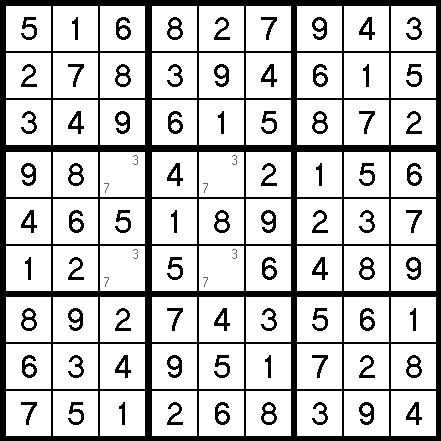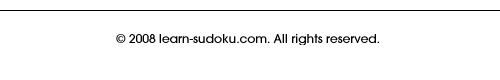## Unique Rectangle

This method is actually a bit controversial, because the logic it uses assumes the fact that the Sudoku puzzle you are working on has only one unique answer.

There is a bit of a disagreement in the Sudoku community on this. While most modern definitions state that a Sudoku puzzle has to have only one answer, a few people claim that a single unique solution is not a requirement. It is true that early Sudoku puzzles were created by hand, and without being tested by computers it was sometimes hard to tell if the puzzle had only one answer. Most people today agree that a puzzle has to be unique to truly be a Sudoku.

The good news is that there is just about a 100% chance the puzzle you are working on does have only one unique answer, but more on that in a minute.

Let me first show you how Unique Rectangle works. Take a look at this example of an invalid puzzle:The puzzle above is almost solved. There are only four cells yet to be filled in. But which ones are 3s and which ones are 7s? You could put a 3 in the upper left cell and solve it from there. But, you could also put an 7 in the upper left and solve it as well. Either way would work, making this puzzle, by most current definitions, invalid.

Ironically, this invalid puzzle is a perfect way for me to illustrate the "Unique Rectangle" principle to you. Notice that the four unsolved cells share exactly two of each kind of "house." That is, they fall in exactly two different rows, exactly two different columns, and exactly two different blocks.

This is how they are able to interchange 3s and 7s — two different numbers being transposed in two different rows, for example, does not upset the balance. Same with two columns and two blocks.

So how can we use this information to solve a real Sudoku? Well, if we can assume the puzzle we are working on had been previously deemed "unique" (which is a pretty safe assumption), then we can also assume there will never be four cells - falling into exactly two rows, two columns, and two blocks - with the same two pencil marks.

Although I'm only guessing, probably well over 99% of the Sudoku puzzles published in books, magazines, and newspapers have one unique answer. How can I be so sure? Because they print the answers as well, and you never see more than one answer!

Even if you didn't really follow everything written above, here is all you really need to know:

Any time you see a rectangle of four unsolved cells, where three of the four cells have the exact same two pencil marks in them, you can remove those pencil marks from the fourth cell completely. Take a look at this puzzle:See the four cells that make up the corners of the blue box? Notice how three of them have only 3 & 5 in them? Well, using our new knowledge, we can safely remove the 3 & 5 from the fourth cell. They just can't possibly be candidates if this is a true Sudoku puzzle.

Pretty cool, huh? The great thing about Unique Rectangle is it is easy to spot — and because of that, you actually see it quite often!

There is just one catch I must stress! This only works when four cells fall into exactly two blocks. Do not get too excited if you see this, because you can't use it:It has to be exactly two blocks.

You don't need to worry about making sure that they fall into exactly two rows and two columns, because the fact that it is a rectangle guarantees that for you!